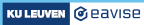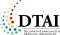3.1. An easy example

### 3.1 An easy example

Once we have defined which situations to consider, we can proceed to distinguish good situations from bad situations. To illustrate, let us start from a simple example. Consider the following small puzzle:

My age is a year more than twice the age of my son. The sum of our ages lies between 70 and 80.

This puzzle talks about the age of two people, so a situation corresponds to a pair of two integers. We can use the following vocabulary:

Here, both functions are nullary, i.e., they take no arguments and just map the empty tuple `()` unto an integer. We call such functions constants since they will have a single fixed value in each structure (in the same way that, e.g., the speed of light is a constant in our universe).

The following are two different structures for this vocabulary.

The set of all structures for this vocabulary is just (isomorphic to) the set `N2` of all pairs of two natural numbers.

In this case, a "good" structure is one that satisfies the clues given by the puzzle. The first clue is this:

`myAge() = 2 * sonsAge() + 1`

The constants (= function without arguments) myAge is followed here by trailing parantheses to denote the integer value to which it maps the empty tuple, rather than the function itself. In other words, in each structure myAge() refers to a specific number. In structure Ages1, the value of myAge() is 31, and in structure Ages2, the value of this same expression myAge() is 10;

The built-in equality operator compares the integer values that the terms myAge() and 2* sonsAge() + 1 have in a specific structure and produces a boolean value from this. In other words, in each structure, this comparison is either true or false: in structure Ages1, it is true, and in Ages2, it is false.

Some terminology: such a boolean expression, which is true or false in each structure, is called a sentence. A theory is a set of sentences. What we have been calling a "good situation" is a structure such that all of the sentences in the theory are true. We also call such a structure a model of the theory.

The following theory expresses all of the clues of the puzzles:

The second sentence of this theory has two "atomic" boolean values produced by the built-in inequality symbols `=<` (less or equal than) and `>=` (greater or equal than), which are then combined into a single boolean value by the "and" operator `&`. The models of this theory, i.e., the "good situations", are the solutions to the puzzle.

It is important to stress that the theory defines what the solutions are, but does not in itself do anything with this knowledge. In fact, this same knowledge can be used for different purposes. Here are some examples of what we can do with this knowledge:

• We can use it to check whether the pair (60, 25) is a solution.
• We can use it to check whether there exists a solution in which "my son" is 25 years old.
• We can use it to find one solution to the puzzle.
• We can use it to find all solutions to the puzzle.
• We can use it to find the largest age that "my son" could have.

### Try it out!

The graph below plots my age and the son's age. You can click anywhere on the graph to see if the point is a solution to the riddle. There are some options below the graph to help you out. Also, feel free to modify the theory and experiment with it! The graph will update automatically using IDP.

There is actually one piece of knowledge that we have not yet expressed in the above theory, namely, that ages are non-negative. In the case of this particular puzzle, we could safely forget about this, because no negative numbers satisfy the two clues anyway. Nevertheless, it might be useful to add this information explicitly anyway, for instance, as a way to safeguard against mistakes and to make our model more robust in case we might wish to change the clues in the future. There are two ways of doing this:

First, we can add it to the theory:

An alternative is to introduce a separate type Age in the vocabulary:

In an associated structure, we can then express that ages are non-negative and bounded by, e.g., 150 years:

Note that this structure interprets the type Age of the vocabulary AgeVoc, but not the two constants myAge and sonsAge. We call this a partial structure for the vocabulary. The possible situations we consider are then no longer all structures for the vocabulary AgeVoc, but only those structures that extend this partial structure. In other words, these are now two possible situations:

The below structure, on the other hand, would no longer be considered a possible situation, because it does not extend AgeStruc.

In general, we often have a clear idea of what the "good situations" are that we want to consider, but we often have a choice whether to build a model that views certain violations, such as a negative age, as a "bad situation" (as when we add the constraint `myAge() >= 0 & sonsAge() >= 0` to the theory) or simply as not even as a "situation" at all (as when we define the type Age as `{0 .. 150}`).

Above, we already made the point that there are often multiple options when choosing a vocabulary for a specific domain. As a further illustration of this point, we can consider another alternative. The puzzle talks about "the age of" two people. Rather than introducing two constants for their two ages, we might instead introduce a unary function ageOf, together with a type Person:

This vocabulary allows us to talk about the ages of arbitrary sets of people: it is a much more widely applicable vocabulary than our original one, at almost no cost in increased complexity. In the context of the puzzle, we are interested in structures that extend this one:

One such structure is the following representation of one of the solutions to the puzzle:

However, this vocabulary does not allow to represent the clues of the puzzle: we cannot write something like `ageOf(Me)`, because the symbol `Me` does not appear in the vocabulary. To be able to represent clues, we must therefore extend our generic vocabulary with two puzzle-specific constants:

We are then interested in structures that extend the following structure.

Using the two new constants, we now can represent the clues of the puzzle:

### Exercise: letter puzzle

In a letter puzzle, each letter corresponds to a digit (from 0 to 9). A sequence of letters, i.e., a word, can then be interpreted as a number, written in standard decimal notation. The goal of the puzzle is to figure out which digits correspond to each letter, given that a given arithmetic relation should hold between these numbers. The following is a famous example:

`LESS + FOOD = DIET`

If you fill in a digit for each letter below, you will see the result of the sum:

 L E S F O D I T ... ... ... ... ... ... ... ...
`… + … =? ….`

Now, let's represent the puzzle by an FO(·) model, which we can use to find solutions to the puzzle.

Start by declaring the appropriate symbols (in the `vocabulary` block) and assigning an appropriate set of values to the types (either in the `structure` block or in the `vocabulary` block). Then add a formula (in the `theory` block) that expresses that the sum must be correct.

Tip: use constants for the letters. Will you allow them to take on any value, or only a specific range?

If you fill in this vocabulary and theory, and click on the "run" button, you can see the calculated solutions. As you will see, there are many solutions. Typically such letter puzzles include two more constraints, namely:

• Different letters also correspond to different digits.
• The starting digit of a number cannot be zero, so for instance the word LESS cannot correspond to 0122, because L cannot be zero.

▶ Click to reveal the solution below, which incorporates these two additional constraints.

###### Made with love (and Hugo) by Joost Vennekens and Simon Vandevelde. :-)